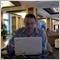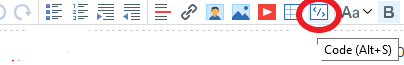# trade timeframe question on backtest7

I have learned the Turtle story and believe the Turtle strategy is a profitable one.

I am trying to build an EA to play with it. I googled and downloaded a demo from Internet  <Deleted>

The demo does not work very well, so I am going to learn mql4 and enhance the demo later.

While I do the backtest with the original demo, I notice that even I use the same strategy on a given currency pair and in the same trading period (say, 1/1/2017-12/31/2017), the result would differ based on different timeframe(M1, M30, H1, H4, Daily). H4 seems to be better in this demo code. I came up with 2 questions:

(1). Why does the test result depends on the test timeframe?

(2). What is the best timeframe to pickup in testing to mimic the real trading in reality?

In my understanding, Turtle strategy is a trend strategy, it usually takes multi days or longer. So I thought the Daily timeframe would be appropriate. However the test results told me the Daily timeframe were typically the worst choice.

Below is the main code:

// External variables

extern int Risk = 1; // 1% of available capital

// Global variables

double  Lots = 0.5;

double  TakeProfit = 100000;

double  MinimumStopLoss = 1000;

double  MagicNumber = 25290428482;

//+------------------------------------------------------------------+

//| Expert initialization function                                   |

//+------------------------------------------------------------------+

int OnInit()

{

Print("==OnInit");

return(INIT_SUCCEEDED);

}

//+------------------------------------------------------------------+

//| Expert deinitialization function                                 |

//+------------------------------------------------------------------+

void OnDeinit(const int reason)

{

}

//+------------------------------------------------------------------+

//| Expert tick function                                             |

//+------------------------------------------------------------------+

void OnTick()

{

int i, ticket, total;

if (Bars < 55) {

Print("==bars less than 55");

return;

}

double highest = High[iHighest(NULL, 0, MODE_HIGH, 55, 1)];

double lowest = Low[iLowest(NULL, 0, MODE_LOW, 55, 1)];

double StopLoss = calculateStopLoss();

// The actual orders

total = OrdersTotal();

if (total < 1) {

if (Bid < lowest) {

// Dont trade if we dont have enough margin

if (AccountFreeMarginCheck(Symbol(), OP_SELL, Lots) <= 0 || GetLastError() == 134) {

return;

}

// create the order

ticket = OrderSend(Symbol(), OP_SELL, Lots, Bid, 3, Bid + StopLoss * Point, Bid - TakeProfit * Point, "my order", MagicNumber, 0, Red);

if (ticket > 0) {

Print("SELL order opened : ",OrderOpenPrice());

}

} else {

Print("Error opening SELL order : ",GetLastError());

return;

}

}

// Dont trade if we dont have enough margin

if (AccountFreeMarginCheck(Symbol(), OP_BUY, Lots) <= 0 || GetLastError() == 134) {

return;

}

// create the order

if (ticket > 0) {

}

} else {

Print("Error opening BUY order : ",GetLastError());

return;

}

}

return;

}

// Check for exit conditions

for (i = 0; i < total; i++) {

continue;

}

if (OrderSymbol() == Symbol()) {

if (Bid < Low[iLowest(NULL, 0, MODE_LOW, 20, 1)]) {

if (!OrderClose(OrderTicket(), OrderLots(), Bid, 3, Green)) {

Print("OrderClose error ", GetLastError());

}

}

} else if (OrderType() == OP_SELL) {

// Sell order

if (Ask > High[iHighest(NULL, 0, MODE_HIGH, 20, 1)]) {

if (!OrderClose(OrderTicket(), OrderLots(), Ask, 3, Green)) {

Print("OrderClose error ", GetLastError());

}

}

}

}

}

}

/**

* Calculate the stop loss based on the account size, risk appetite and lot size

*/

double calculateStopLoss()

{

return MathMax(MinimumStopLoss, NormalizeDouble(AccountFreeMargin() * Risk / 100 / (Lots * MarketInfo(Symbol(), MODE_TICKVALUE)), 0));

}Moderator
114323